# How to Simulate Financial Portfolios with PythonLearn how to simulate financial portfolios with Python. An application of Multidimensional Geometric Brownian Motion (GBM), GBM is a powerful method to implement a simple and worthwhile model for market simulation. This article aims to model one or more stock prices in a portfolio using the multidimensional Geometric Brownian Motion model.

In an increasingly globalised world, the financial markets are constantly influenced by a rising number of factors: news, politics, sport… and above all the market itself. Many scientists are trying to abstract and model these interactions between events outside and inside the financial market. However, in quantitative finance it’s hard to develop a representative model of the actual market. Nevertheless, a valuable model for market simulation is needed to tackle problems such as:

• Option Pricing;
• Portfolio valuation;
• Hedging strategies;
• Sensitivity analysis;
• Uncertain future cash flows calculation.

In short, efficiently simulating as many scenarios as possible helps us to reduce the inconvenience caused by market uncertainty. Below I present a powerful method to implement a simple and worthwhile model for market simulation.

Price trend of single stock can be shaped as a stochastic process, known as Geometric Brownian Motion (GBM) model. However, for a portfolio consisting of multiple corporate stocks, we need an expansion of the GBM model. This article aims to model one or more stock prices in a portfolio using the multidimensional Geometric Brownian Motion model.

### Summary

1. Introduction
2. Theory and Algorithm
3. Implementation in Python
4. Portfolio Simulation Examples
5. Conclusion

### Introduction

Even though we can calculate a bullet’s trajectory with pinpoint accuracy, predicting a market trend seems to be impossible. At least to the present day.

This is due to the stochastic nature of the financial market presenting features that make it a perfect example of complex system. In fact complex systems have a behavior that is intrinsically difficult to shape because of the interactions among their parts (in a physico-mathematical sense). In complex systems collective behavior of their parts causes the appearance of features which can hardly be deduced from the single parts properties, if not at all.

“The whole is something besides the parts.”

The complexity of financial market derives from the presence of a high level of correlation between its parts. The nonlinear nature of the interactions is reflected on the cause and effect processes until it produces unpredictable events. For this reason, the nature and the dynamic characteristics of the correlations among their actions are key aspects of financial market complexity.

Then it is important to build a coherent and as realistic as possible market model so that the study of this characteristic could improve the economic forecast and the shaping of the composite financial scales, such as share portfolios.

### Theory and Algorithm

One-dimensional

A Geometric Brownian motion is a continuous-time stochastic process. More rigorously, Geometric Brownian motion process is specified through an stochastic differential equation (SDE) of the form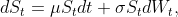where W is a Brownian motion, and _µ _and σ are constants representing respectively the percentage drift and the percentage volatility.

The above SDE has the analytic solution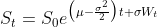that provides a simple recursive procedure for simulating values of S at every instant of temporal discretization: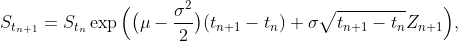for n = 0, 1, …, N -1, with _Z _independent standard normals.

Multiple Dimensions

A multidimensional geometric Brownian motion can be specified through a system of SDEs. Assuming you want to simulate a portfolio of d stocks, the system takes the following form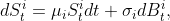with_ i = 1, . . . , d._

The information relating to the correlations between the stocks is contained within the Brownian motions, in fact we have that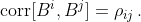If we define a _d x d _matrix Σ by setting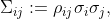in a convenient abuse of terminology, we refer to Σ as covariance matrix; although the covariances are given by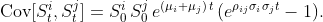Recall that a Brownian motion with mean 0 and covariance matrix Σ can be represented as AW with W a standard Brownian motion and A any matrix for which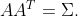We may apply this property and rewrite SDE’s system asthis representation leads to a simple algorithm for simulating multidimensional GBM: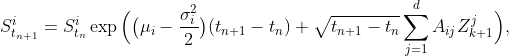for i = 1, . . . , d and n = 0, …, N -1, where Z is a standard normal random vector.

Note: choosing _A _to be the Cholesky factor of Σ can reduce the number of multiplications and additions required at each step.

For more details see the references .

### Implementation in Python

We use the numpy package and its vectorization properties to make the program more compact, easier to read, maintain and faster to execute.

We define a function to simulate a multidimensional GBM given the parameters. In addition to the model parameters we have chosen to insert a parameter relating to the randomization seed so as to be able to repeat a simulation with the same results.

We build an array of size (number of stocks, number of increments) containing the paths of all simulated stocks.

``````## Asset Path
import numpy as np

def GBMsimulator(seed, So, mu, sigma, Cov, T, N):
"""
Parameters

seed:   seed of simulation
So:     initial stocks' price
mu:     expected return
sigma:  volatility
Cov:    covariance matrix
T:      time period
N:      number of increments
"""

np.random.seed(seed)
dim = np.size(So)
t = np.linspace(0., T, int(N))
A = np.linalg.cholesky(Cov)
S = np.zeros([dim, int(N)])
S[:, 0] = So
for i in range(1, int(N)):
drift = (mu - 0.5 * sigma**2) * (t[i] - t[i-1])
Z = np.random.normal(0., 1., dim)
diffusion = np.matmul(A, Z) * (np.sqrt(t[i] - t[i-1]))
S[:, i] = S[:, i-1]*np.exp(drift + diffusion)
return S, t``````

## Python For Data Science | Python For Data Analysis

Python for Data Science, you will be working on an end-to-end case study to understand different stages in the data science life cycle. This will mostly deal with "data manipulation" with pandas and "data visualization" with seaborn. After this, an ML model will be built on the dataset to get predictions. You will learn about the basics of the sci-kit-learn library to implement the machine learning algorithm.

## Basic Data Types in Python | Python Web Development For Beginners

In the programming world, Data types play an important role. Each Variable is stored in different data types and responsible for various functions. Python had two different objects, and They are mutable and immutable objects.

## Hire Python Developers

Are you looking for experienced, reliable, and qualified Python developers? If yes, you have reached the right place. At **[HourlyDeveloper.io](https://hourlydeveloper.io/ "HourlyDeveloper.io")**, our full-stack Python development services...

## Applied Data Science with Python Certification Training Course -IgmGuru

Master Applied Data Science with Python and get noticed by the top Hiring Companies with IgmGuru's Data Science with Python Certification Program. Enroll Now

## Data Science With Python | Python For Data Science | Data Science For Beginners

This Data Science with Python Tutorial will help you understand what is Data Science, basics of Python for data analysis, why learn Python, how to install Python, Python libraries for data analysis, exploratory analysis using Pandas, introduction to series and dataframe, loan prediction problem, data wrangling using Pandas, building a predictive model using Scikit-Learn and implementing logistic regression model using Python.